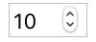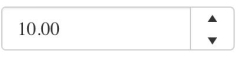In the previous episode, you learned how the `MaskedTextBox` is used to format user input. In this episode, you will learn how the `NumericTextBox` is used to format numeric input.

The `NumericTextBox` is a form field that restricts input to numbers and has a button to increment and decrement its values. The purpose of this component is to provide more control over formatting the data so you can achieve greater accuracy. For example, currencies come in different denominations and this affects how many significant digits the input should have and how to round. Coming up, you will see a comparison of the HTML number input type and the Kendo UI `NumericTextBox`.

## HTML Number Input

To create a numeric textbox using plain HTML, you create an input element and set the `type` attribute to `number`. By default, the input will increment and decrement values by one. If a number other than an integer is entered, the values will round to the nearest integer. You can further restrict the input by adding attributes to the element. The `max` attribute sets the maximum value for the field. The `min` attribute sets the minimum value for the field. And the `step` attribute sets the intervals of increase and decrease. The following example can accept numbers between 0 and 100 and will increase and decrease the values by 10.``<input id="textbox" type="number" min="0" max="100" step="10">``

The step also affects how rounding is calculated and the number of decimal places used. For example, if you use a step of .1 the input entered will be rounded to the nearest tenths place. If you use a step of .01 the input will be rounded to the nearest hundredths place. But what if you don't want the step to correspond to the number of decimal places? Using our money example, if the input field accepts US dollars we may allow the user to enter a number with up to two decimal places. However, we may want the step to be one dollar because it is not useful for the interval to be one cent. For this level of control, we need the `NumericTextBox`.

## Kendo UI NumericTextBox

Using the same code from above, we can transform our input field into a Kendo UI `NumericTextBox` with the following:``````<input id="textbox" type="number" min="0" max="100" step="10">
<script>
\$('#textbox').kendoNumericTextBox();
});
</script>``````

The `min`, `max`, and `step` can be set in the attributes of the input as we did in the previous examples or they can be set in the component's API. This is how we would configure these properties inside the code:

``````<input id="textbox">
<script>
\$('#textbox').kendoNumericTextBox({
min: 0,
max: 100,
step: 10
});
});
</script>``````

To change the format of the input, you set the `format` option. The format is what the widget displays when it is not focused. After entering a number, the format will appear when the mouse loses focus on the input field. Possible values for the format are `n` (number), `c` (currency), `p` (percentage), and `e` (exponent). The number of decimals can be also set in the format field by adding a digit to the end of the format.

For example, to format a number with zero decimals is `n0`. To format a currency with three decimals is `c3`. The `decimals` property can be used to configure what the widget shows when it is focused. If the `format` is n2 and `decimals` is 3, the widget will use a precision of two decimals when the field loses focus. However, when the field is in focus, it will use a precision of three decimals.

## Conclusion

Both the HTML number input and the Kendo UI `NumericTextBox` allow you to restrict data with minimum and maximum values and control the step. However, the `NumericTextBox` also lets you define the precision of the numbers and format other number types. Furthermore, if there is a format you would like to use that doesn't conform to one of the predefined types, you can create a custom format. This is useful when you want to use symbols to represent other kinds of data like weight or volume. It is also useful when you want to customize the number of digits in the input. If the value of the input is a binary number you could make sure the input is a certain length by padding the beginning with zeros. In the next lesson, we will continue our exploration of inputs with the `DatePicker` component.

## Try Out the NumericTextBox for Yourself

Want to start taking advantage of the Kendo UI jQuery NumericTextBox, or any of the other 70+ ready-made Kendo UI components, like Grid or Scheduler? You can begin a free trial of Kendo UI today and start developing your apps faster.

## Angular, React, and Vue Versions

Looking for a NumericTextBox to support specific frameworks? Check out the NumericTextBox for Angular, the NumericTextBox for React, or the NumericTextBox for Vue.

## Resources